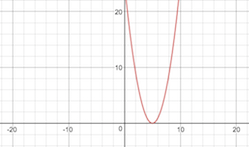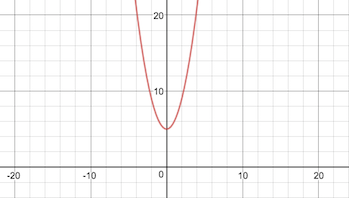# Transformations of Quadratic Functions

An error occurred trying to load this video.

Try refreshing the page, or contact customer support.

Coming up next: Parabolas in Standard, Intercept, and Vertex Form

### You're on a roll. Keep up the good work!

Replay
Your next lesson will play in 10 seconds
• 0:04 Quadratic Functions
• 0:32 Graphing Quadratic Functions
• 1:06 Transformation
• 3:54 Lesson Summary

Want to watch this again later?

Timeline
Autoplay
Autoplay
Speed

#### Recommended Lessons and Courses for You

Lesson Transcript
Instructor: Stephanie Matalone

Stephanie taught high school science and math and has a Master's Degree in Secondary Education.

In this lesson, we will not only go over the basic definition of a quadratic function, we will also talk about transformations of those functions. In other words, we will discuss how to move the graph around by changing the formula.

You stand in your backyard and throw a ball into the air. It makes a nice arc and then comes back down to the ground. That pretty shape you just made looks exactly like the graph of a quadratic function! Quadratic functions are second order functions, which means the highest exponent for a variable is two. They're usually in this form: f(x) = ax2 + bx + c. One thing to note about that equation is that the coefficient a cannot be equal to zero.

## Graphing Quadratic Functions

Quadratic functions can be graphed just like any other function. The neat thing about these is that they will always graph into a curved shape called a parabola. Parabolas are u-shaped and can be upside down depending on the numbers in the equation.

Let's look at the parent function of a quadratic: f(x) = x2. If we compare this to the usual form of f(x) = ax2 + bx + c, we can see that a = 1, b = 0, and c = 0. When we graph this parent function, we get our typical parabola in an u-shape.

## Transformation

Let's say you took a step to the left and threw the ball higher in your backyard. You just transformed your parabola! We can transform graphs by shifting them, flipping them, stretching them, or shrinking them. We can do this by changing the equation of the graph.

The first type of transformations we will deal with are called shifts. This means we are moving the graph horizontally to the left or right or vertically up or down. Let's say we want to move our parent graph of f(x) = x2 to the right five units. To do this, we have to subtract five from the x value inside parentheses like so: f(x) = (x - 5)2. Any shifts to the right will be completed through subtracting number inside the parentheses, while any shifts to the left will done be by adding a number inside the parentheses.If you want to shift the graph up five, you will add five to x, but this time, you do not need parentheses, or you can go outside of them: f(x) = x2 + 5 or f(x) = (x2) + 5. Any vertical shifts up will be done by adding a number outside of the parentheses, while any vertical shifts down will come from subtracting a number outside of the parentheses.If you want to change the width of your graph, you can do so in the vertical or horizontal direction. To compress or stretch vertically, you will multiply the entire equation by a number. If that number is greater than one, the graph will stretch. If that number is between 0 and 1, that graph will compress. Think about the graph being pushed on from above and below and being compressed towards the x-axis. For example, the function f(x) = 1/4(x2) will compress vertically. In other words, the graph will get wider.

To unlock this lesson you must be a Study.com Member.

### Register to view this lesson

Are you a student or a teacher?

### Unlock Your Education

#### See for yourself why 30 million people use Study.com

##### Become a Study.com member and start learning now.
Back
What teachers are saying about Study.com

### Earning College Credit

Did you know… We have over 160 college courses that prepare you to earn credit by exam that is accepted by over 1,500 colleges and universities. You can test out of the first two years of college and save thousands off your degree. Anyone can earn credit-by-exam regardless of age or education level.